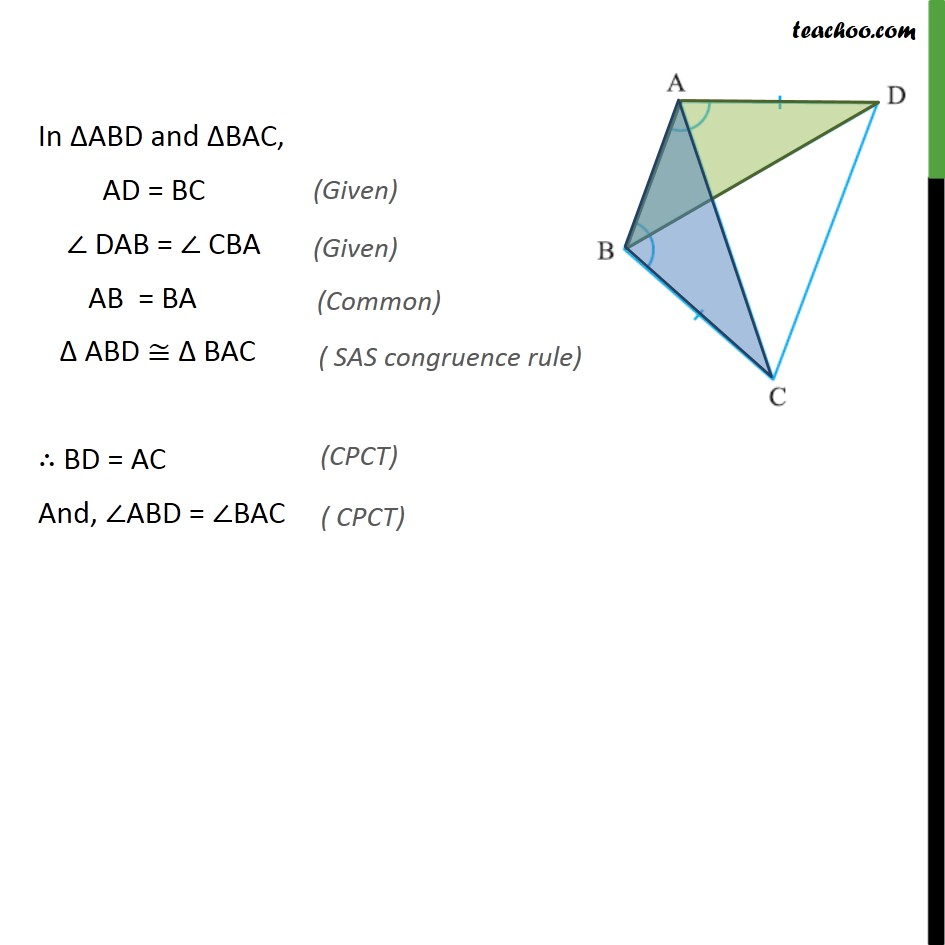Ex 7.1

Chapter 7 Class 9 Triangles
Serial order wiseGet live Maths 1-on-1 Classs - Class 6 to 12

### Transcript

Ex 7.1, 2 ABCD is a quadrilateral in which AD = BC and DAB = CBA (See the given figure). Prove that (i) ABD BAC (ii) BD = AC (iii) ABD = BAC. Given: AD = BC and DAB = CBA To prove: (i) ABD BAC , (ii) BD = AC , (iii) ABD = BAC Proof: In ABD and BAC, AD = BC DAB = CBA AB = BA ABD BAC BD = AC And, ABD = BAC